# Stress Concentrations

Stress concentrations are deviations from the nominal stress on a part. What causes a stress concentration is an abrupt change in the flow of stress through a part. This abrupt change can cause a higher stress or a lower stress by a certain factor in comparison to the nominal stress.

A good analogy for stress concentrations is a river. When the flow of water in a river comes across an object such as a rock the flow of the water will change around the rock. However, if the water is far enough away from the rock then it will not be affected as much or the flow will remain the nominal.

Below there are images of how a stress concentration would apply for a plate that is under an axial load. One image is plate with a hole in it. Another is the plate with slots in it, and the remaining one is a larger plate connected to a smaller plate with fillets at the connection point.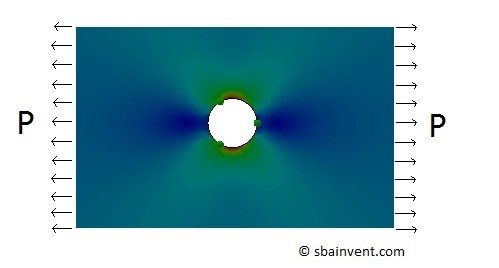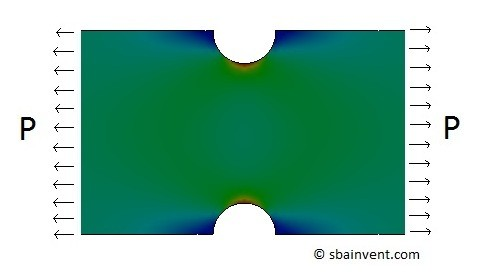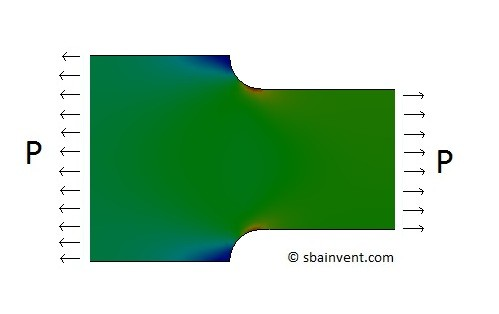Notice in all three figures where there is an abrupt change to alter the stress flow the stress changes. If the transition is made smoother then stress concentration will become lower, while a more rapid change will result in a higher stress concentration. Basically, for example, if the radius for the fillet is larger it will have lower stress value then a smaller radius or no radius applied at all. The same holds true for holes and the slots. Refer to the figure below.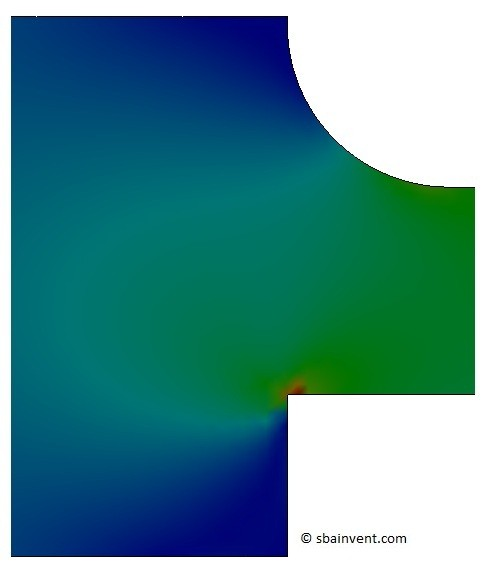Notice from the figure above that the corner that doesn’t have fillet has a red hot spot due to a high stress. While the large fillet that has a smooth transition has a lower stress concentration and the stress values merge together much more easily. Taking this in consideration when designing parts, it is important to keep stress concentrations in mind, and unless it isn’t possible to do so, all corners should be rounded if that application is dealing with high stresses.

Another thing to consider when you have something like two holes close to each other, how will that effect the stress concentration. What will happen is the stress concentrations will interact with one another and will add together. Refer to the figure below.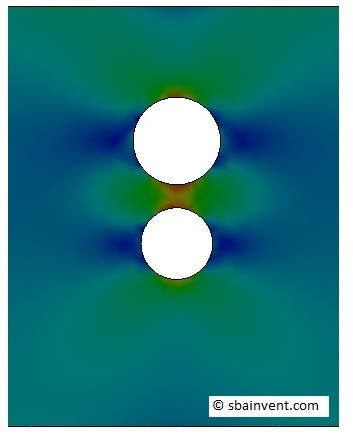## Calculating Stress Concentrations

To calculate stress concentrations it easier and much more accurate to use a finite element program then trying to calculate a stress concentration by hand. For example I used SolidWorks Simulation to generate all of the figures above. However, if an FEA program is not available you can use graphs or advanced mathematical calculations to estimate basic stress concentrations. All of this can be found in most strength of materials text books in great detail. However, I have provided a graph below that can be used to estimate the stress concentration at a hole, a slot, and a fillet for plates that are under an axial load.